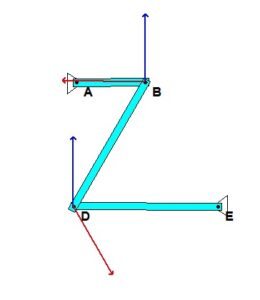# Homework H.2.JDiscussionThe motion of this four-bar mechanism is quite complicated. However, we can make some sense out of the velocities of points B and D by using instant centers (ICs). For a given position of the mechanism, can you visualize the location of the IC for link BD? And from this location, do the directions for the velocities for points B and D make sense?

HINTS:
You are asked to perform the complete kinematical analysis for the mechanism for the position shown below.

Velocity
For the velocity analysis, you are encouraged to use the IC center approach. Where is the IC for link BD located? What does the location of this IC say about the angular velocity of link BD? Use that result to determine the angular velocity of link DE.

Acceleration
This is a standard kinematics of a mechanism problem. For this, consider the following steps:

1. Write down the acceleration of point B using link AB.
2. Write down the acceleration expression for D using link DE.
3. Write down the acceleration expression for B using link DB and your result for the acceleration of D from Step 2.
4. Equate the expressions for the acceleration of B in Steps 1 and 3 above. From this, solve for the angular accelerations of links BD and DE.## 4 thoughts on “Homework H.2.J”

1.jone1871 says:

The problem statement states that omega is counter-clockwise, but the diagram shows clockwise. Which one should we use to solve?

1.CMK says:

Use the figure. If you have already worked it the other way, that is fine also.

2.Ethan Chu says:

Are we expected to leave our ans in terms of Woa and L?

1.Hongcheng Tao says:

Yes (ω_AB)